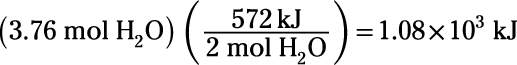##### Chemistry For Dummies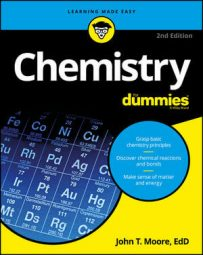By calculating the enthalpy change in a chemical reaction, you can determine whether the reaction is endothermic or exothermic. Chemical reactions transform both matter and energy. Though chemical equations usually list only the matter components of a reaction, you can also consider heat energy as a reactant or product. When chemists are interested in heat flow during a reaction (and when the reaction is run at constant pressure), they may list an enthalpy change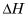to the right of the reaction equation. At constant pressure, heat flow equals enthalpy change: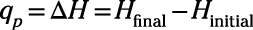If the enthalpy change listed for a reaction is negative, then that reaction releases heat as it proceeds — the reaction is exothermic (exo- = out). If the enthalpy change listed for the reaction is positive, then that reaction absorbs heat as it proceeds — the reaction is endothermic (endo- = in). In other words, exothermic reactions release heat as a product, and endothermic reactions consume heat as a reactant. The sign of thetells you the direction of heat flow, but what about the magnitude? The coefficients of a chemical reaction represent molar equivalents, so the value listed for therefers to the enthalpy change for one mole equivalent of the reaction. Here's an example: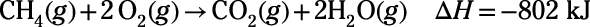This reaction equation describes the combustion of methane, a reaction you might expect to release heat. The enthalpy change listed for the reaction confirms this expectation: For each mole of methane that combusts, 802 kJ of heat is released. The reaction is highly exothermic. Based on the stoichiometry of the equation, you can also say that 802 kJ of heat is released for every 2 mol of water produced.

So reaction enthalpy changes (or reaction "heats") are a useful way to measure or predict chemical change. But they're just as useful in dealing with physical changes, like freezing and melting, evaporating and condensing, and others. For example, water (like most substances) absorbs heat as it melts (or fuses) and as it evaporates. Here are the molar enthalpies for such changes:

• Molar enthalpy of fusion: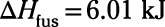• Molar enthalpy of vaporization: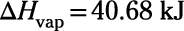The same sorts of rules apply to enthalpy changes listed for chemical changes and physical changes. Here's a summary of the rules that apply to both:
• The heat absorbed or released by a process is proportional to the moles of substance that undergo that process. For example, 2 mol of combusting methane release twice as much heat as 1 mol of combusting methane.

• Running a process in reverse produces heat flow of the same magnitude but of opposite sign as running the forward process. For example, freezing 1 mol of water releases the same amount of heat that is absorbed when 1 mol of water melts.

Try an example: here is a balanced chemical equation for the oxidation of hydrogen gas to form liquid water, along with the corresponding enthalpy change: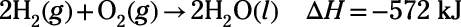How much electrical energy must be expended to perform electrolysis of 3.76 mol of liquid water, converting that water into hydrogen gas and oxygen gas?

First, recognize that the given enthalpy change is for the reverse of the electrolysis reaction, so you must reverse its sign from –572 kJ to 572 kJ. Second, recall that heats of reaction are proportional to the amount of substance reacting (2 mol of H2O in this case), so the calculation is